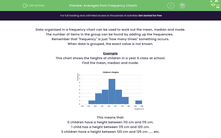# Averages from Frequency Charts

In this worksheet, students read grouped data from frequency charts in order to work out the mean, median and mode.Key stage:  KS 3

Curriculum topic:   Statistics

Curriculum subtopic:   Understand Variables, Representation, Measures and Spread

Difficulty level:#### Worksheet Overview

Data organised in a frequency chart can be used to work out the mean, median and mode.

The number of items in the group can be found by adding up the frequencies.

Remember that "frequency" is just "how many times" something occurs.

When data is grouped, the exact value is not known.

Example

This chart shows the heights of children in a year 6 class at school.

Find the mean, median and mode.This means that:

0 children have a height between 110 cm and 115 cm.

1 child has a height between 115 cm and 120 cm.

3 children have a height between 120 cm and 125 cm ..... etc.

Mean

We have to estimate the mean by using the midpoint value in each class.

These are 112.5, 117.5, 122.5, 127.5, 132.5, 137.5, 142.5, 147.5 and 152.5 cm

The total estimated height of all the children is    (1 x 117.5) + (3 x 122.5) + (4 x 127.5) + (7 x 132.5) + (4 x 137.5) + (2 x 142.5) + (1 x 152.5) = 2910 cm

There are 1 + 3 + 4 + 7 + 4 + 2 + 1 = 22 children in the class.

Mean height = 2910 ÷ 22 = 132.3 cm (1 dp)

Median

There are 22 children, so the "middle person" will be the 11th and 12th.

The 11th and 12th people fall into the 130 cm to 135 cm class

The median class is 130 cm to 135cm.

Mode

This is the class with the highest frequency.

The modal class  130 cm to 135cm.

### What is EdPlace?

We're your National Curriculum aligned online education content provider helping each child succeed in English, maths and science from year 1 to GCSE. With an EdPlace account you’ll be able to track and measure progress, helping each child achieve their best. We build confidence and attainment by personalising each child’s learning at a level that suits them.

Get started